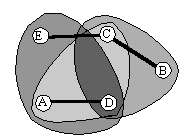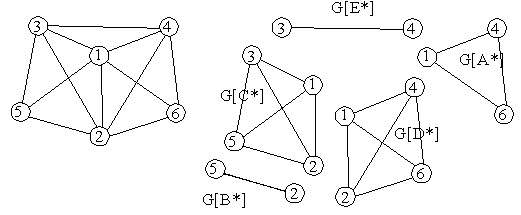### Examples for the notion of duality

A convenient way to think of duals is by the incidence matrix of H, where the columns are labeled by the elements of A and the rows by the elements of V, and there is a `1' (or `*`, as in the figures) in row x and column a if aSx, otherwise there is a `0`. Thus the rows `correspond' to the hyperedges of H.

We give a hypergraph by its list of hyperedges, as Venn diagram, and by its incidence matrix.

 S1={ A, C, D, } S2={ B, C, D, } S3={ C, E, } S4={ A, D, E, } S5={ B, C, } S6={ A, D, }A B C D E 1 * 0 * * 0 2 0 * * * 0 3 0 0 * 0 * 4 * 0 0 * * 5 0 * * 0 0 6 * 0 0 * 0

By transposing the matrix, the roles of columns and vertices are interchanged. The dual H* is the hypergraph whose incidence matrix equals the transpose of the incidence matrix of H. Now the dual of the hypergraph above is described as follows:
 1 2 3 4 5 6 A * 0 0 * 0 * B 0 * 0 0 * 0 C * * * 0 * 0 D * * 0 * 0 * E 0 0 * * 0 0
 A*={ 1, 4, 6, } B*={ 2, 5, } C*={ 1, 2, 3, 5, } D*={ 1, 2, 4, 6, } E*={ 3, 4, }The intersection graph of the first hypergraph isBack to the start page for intersection graphs.

Erich Prisner